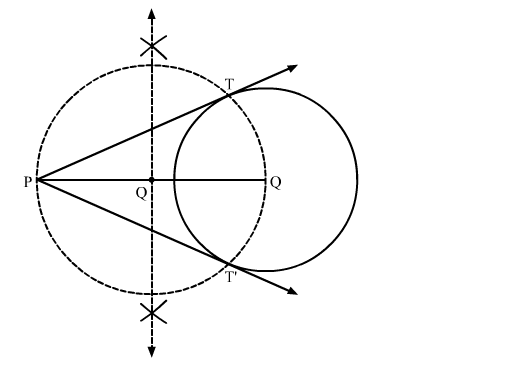# Draw two tangents to a circle of radius 3.5 cm from a point P`
Question:

Draw two tangents to a circle of radius 3.5 cm from a point P at a distance of 6.2 cm from its centre.

Solution:

Steps of Construction

Step 1. Draw a circle with O as centre and radius 3.5 cm.

Step 2. Mark a point P outside the circle such that OP = 6.2 cm.

Step 3. Join OP. Draw the perpendicular bisector XY of OP, cutting OP at Q.

Step 4. Draw a circle with Q as centre and radius PQ (or OQ), to intersect the given circle at the points T and T'.

Step 5. Join PT and PT'.Here, PT and PT' are the required tangents.